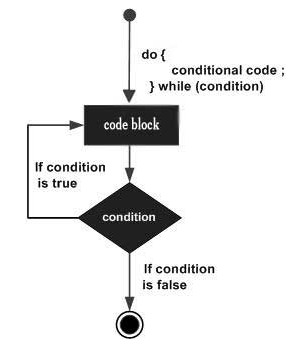×

Search anything:

# do while loop control statement

#### control statement loop while loop do while loopGet this book -> Problems on Array: For Interviews and Competitive Programming

A do-while loop is similar to a while loop, except the fact that it is guaranteed to execute at least one time. The do-while loop checks its condition at the bottom of the loop after one time execution of do.

Syntax

``````
do
{
statement(s);
}
while( condition );
``````

The condition appears at the end of the loop, so the statement(s) in the loop executes once before the condition is tested.

### Flow DiagramIf the condition is true, the flow of control jumps back up to do, and the statement(s) in the loop executes again. This process repeats until the given condition becomes false.
If the condition becomes false statement following the while statement will be executed.

## Example

write a program to print 'n' until its value is less than 20 using do-while.

``````
int main ()
{
/* local variable definition */
int n = 10;

/* do loop execution */
do {
printf("value of n: %d\n", n);
n = n + 1;
}while( n < 20 );

return 0;
}
``````

Output

``````
value of n: 10
value of n: 11
value of n: 12
value of n: 13
value of n: 14
value of n: 15
value of n: 16
value of n: 17
value of n: 18
value of n: 19
``````#### Tanya Anand

Final Year Undergrad (2020) | CSE | NIT Hamirpur | Intern at OpenGenus

do while loop control statement